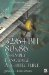Appendix A: Data Structure DefinitionsWhen dealing with three-dimensional transformation, data structures are needed to contain the data type. The data structures listed here are discussed in terms of this book.

Integer 2D Point

This is mostly used within a 2D game but has uses within a 3D game. It is used for specifying a starting point within a bitmap image with the origin {0,0} located in the upper-left corner. This is primarily used as the starting point of a texture within a bitmap image. It is also used in relationship to the anchor point of a font baseline so as where to position it within an image or two-triangle quad polygon tile.

typedef struct iPosType {   int   x;   int   y; } iPos;

Integer 2D Size

Normally bitmaps range from 0 to w1 for width and 0 to h1 for height and are never negative. With that in mind, only unsigned arguments are used.

typedef struct iSizeType {   uint  w;      // Width   uint  h;      // Height } iSize;

Integer Rectangle

Some rectangle structures are designed with a field definition such as {x1,y1,x2,y2}, but any movement has to be translated with the modification of four coordinates. With the following structure {x,y,w,h}, only the two fields {x,y} need to be modified.

typedef struct iRectType {   int   x;      // X coord.   int   y;      // Y coord.   uint  w;      // Width   uint  h;      // Height } iRect;

3D Vector (Integer)

typedef struct iVector3DType {   int   x;   int   y;   int   z; } iVector3D;

3D Quad Vector (Integer)

typedef struct iQVector3DType {   int   x;   int   y;   int   z;   int   w; } iQVector3D;

3D Vector (Floating Point)

typedef struct vmp3DVector {   float x;   float y;   float z; } vmp3DVector;           vmp3DVector point =   {0.0, 0.0, 0.0};   vmp3DVector vector = {1.0, 2.0, 3.0};

3D Quad Vector (Floating Point)

typedef struct vmp3DQVector {   float x;   float y;   float z;   float w; } vmp3DQVector;         vmp3DQVector point =   {0.0, 0.0, 0.0, 0.0}; vmp3DQVector vector = {1.0, 2.0, 3.0, 0.0};

Quaternion (Single-precision Floating-point)

typedef struct vmpQuat {   float x;   float y;   float z;   float w; } vmpQuat;32/64-Bit 80x86 Assembly Language Architecture
ISBN: 1598220020
EAN: 2147483647
Year: 2003
Pages: 191

Similar book on Amazon